# 线性回归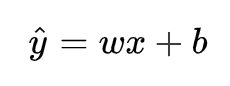y^为预测值，自变量x和因变量y是已知的，而我们想实现的是预测新增一个x，其对应的y是多少。

# 目标/损失函数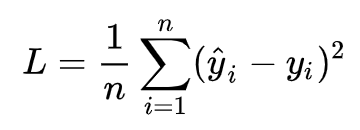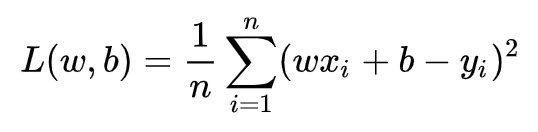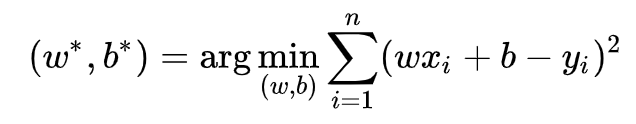# 求解方式有两种：

## 1）最小二乘法(least square method)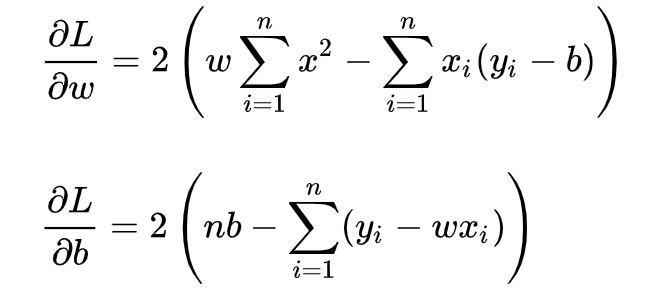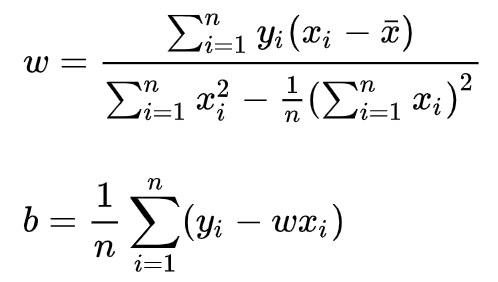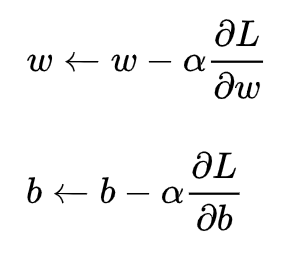# 最小二乘法公式推导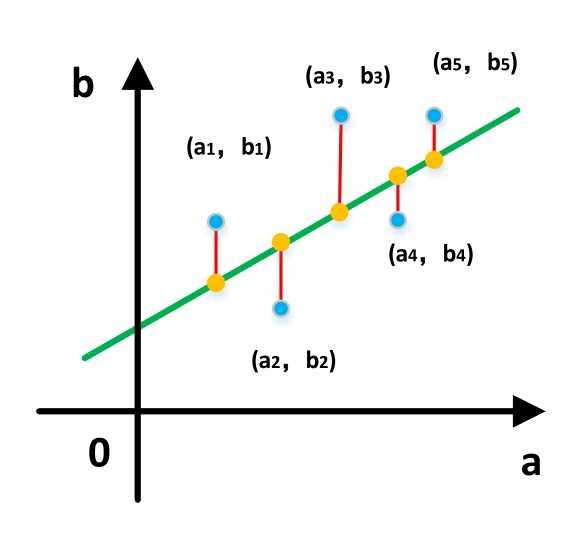# 推导过程

y=a+bx

di=yi−(a+bxi)

## 4 设D为差方和（为什么要取平方前面已说，防止正负相互抵消）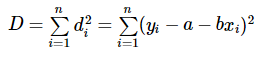## 5 根据一阶导数等于0，二阶大于等于0（证明略）求出未知参数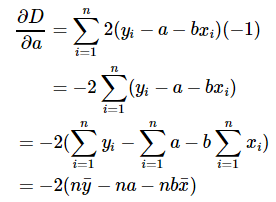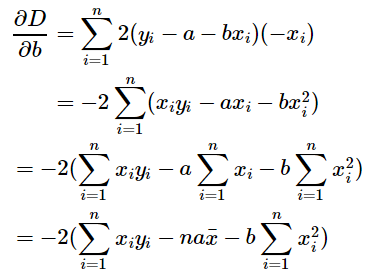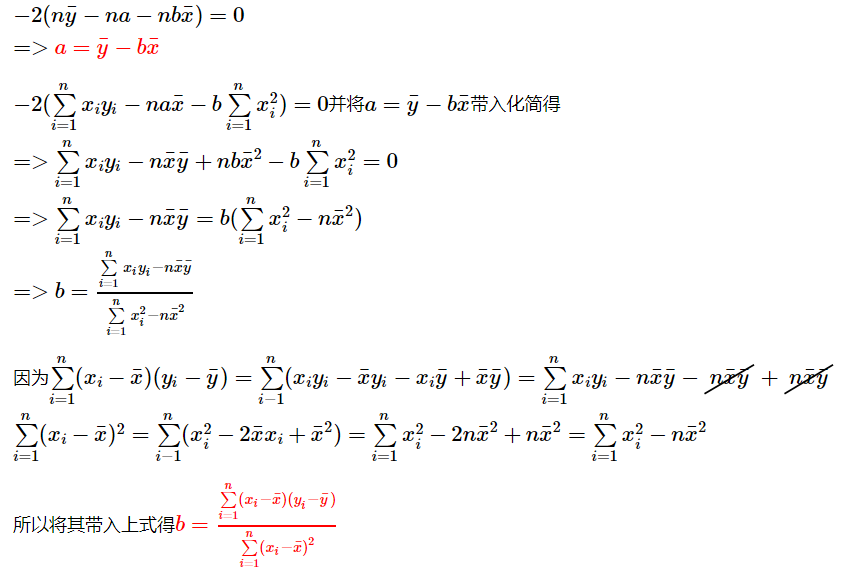ps: 知道了公式之后代码实现起来就会非常简单。

# java 实现

package com.github.houbb.gene.math;

import java.util.Arrays;
import java.util.List;

/**
* @author binbin.hou
* @since 1.0.0
*/
public class LinerRegression {

private static class Point {
private double x;
private double y;

public Point(double x, double y) {
this.x = x;
this.y = y;
}

public double getX() {
return x;
}

public double getY() {
return y;
}
}

public void regression(List<Point> list) {
//1. 計算 x/y 的平均值
double[] means = calcMean(list);
double xMean = means;
double yMean = means;

// 假设方程为 y = a + bx
double sum = 0;
double sumDiv = 1;
for(Point point : list) {
double x = point.getX();
double y = point.getY();

sum += (x - xMean) * (y - yMean);
sumDiv += (x - xMean) * (x - xMean);
}

double b = sum / sumDiv;
double a = yMean - b * xMean;

System.out.println("线性回归方程：y = " + a + " + ("+ b + ")*x");
}

/**
* 計算 x/y 的平均值
* @param list 列表
* @return 结果
*/
private double[] calcMean(List<Point> list) {
double xSum = 0;
double ySum = 0;
for(Point point : list) {
xSum += point.getX();
ySum += point.getY();
}

// 平均值
double xMean = xSum / list.size();
double yMean = ySum / list.size();
return new double[]{xMean, yMean};
}

public static void main(String[] args) {
List<Point> list = Arrays.asList(new Point(1,1),
new Point(2,2), new Point(3,3), new Point(4,4));

LinerRegression regression = new LinerRegression();
regression.regression(list);

//        y = 0.4166666666666665 + (0.8333333333333334)*x
System.out.println(0.4166666666666665 + 0.8333333333333334 * 2);
}

}


# 参考资料

 [机器学习 算法笔记- 线性回归（Linear Regression）](https://www.cnblogs.com/geo-will/p/10468253.html)